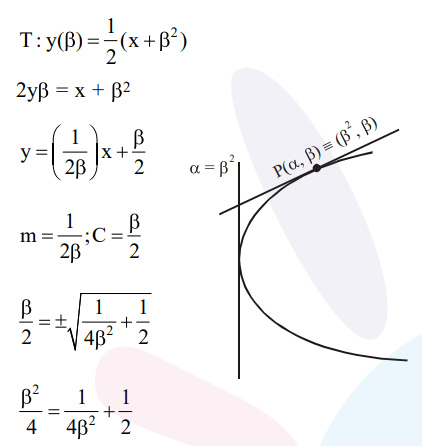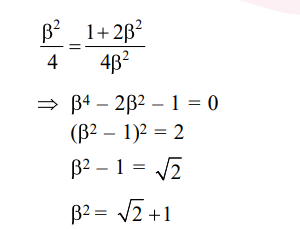# If the tangent to the parabola`
Question:

If the tangent to the parabola $\mathrm{y}^{2}=\mathrm{x}$ at a point $(\alpha, \beta),(\beta>0)$ is also a tangent to the ellipse, $x^{2}+2 y^{2}=1$, then $\alpha$ is equal to :

1. $2 \sqrt{2}+1$

2. $\sqrt{2}-1$

3. $\sqrt{2}+1$

4. $2 \sqrt{2}-1$

Correct Option: , 3

Solution: